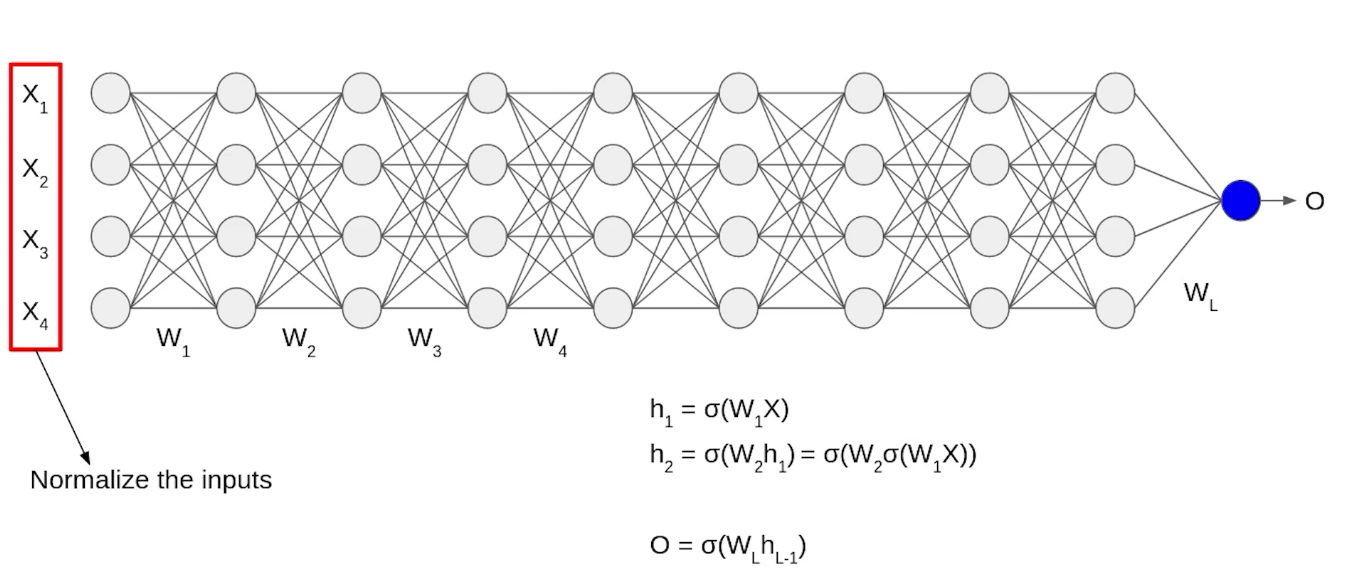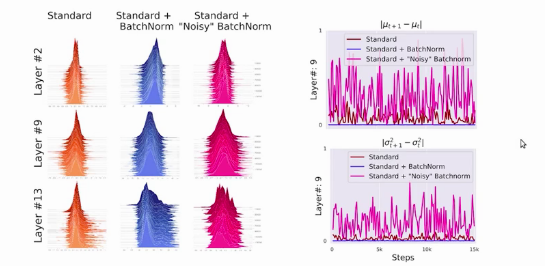Shipra Saxena — Published On March 9, 2021 and Last Modified On August 3rd, 2023

## Objective

• Learn how to improve the neural network with the process of Batch Normalization.
• Understand the advantages batch normalization offers.

## Introduction

One of the most common problems of data science professionals is to avoid over-fitting. Have you come across a situation when your model is performing very well on the training data but is unable to predict the test data accurately. The reason is your model is overfitting. The solution to such a problem is regularization.

Note: If you are more interested in learning concepts in an Audio-Visual format, We have this entire article explained in the video below. If not, you may continue reading.

The regularization techniques help to improve a model and allows it to converge faster. We have several regularization tools at our end, some of them are early stopping, dropout, weight initialization techniques, and batch normalization. The regularization helps in preventing the over-fitting of the model and the learning process becomes more efficient.

Here, in this article, we are going to explore one such technique, batch normalization in detail.

## What is Batch Normalization?

Before entering into Batch normalization let’s understand the term “Normalization”.

Normalization is a data pre-processing tool used to bring the numerical data to a common scale without distorting its shape.

Generally, when we input the data to a machine or deep learning algorithm we tend to change the values to a  balanced scale. The reason we normalize is partly to ensure that our model can generalize appropriately.

Now coming back to Batch normalization, it is a process to make neural networks faster and more stable through adding extra layers in a deep neural network. The new layer performs the standardizing and normalizing operations on the input of a layer coming from a previous layer.

But what is the reason behind the term “Batch” in batch normalization? A typical neural network is trained using a collected set of input data called batch. Similarly, the normalizing process in batch normalization takes place in batches, not as a single input.

Let’s understand this through an example, we have a deep neural network as shown in the following image.

Initially, our inputs X1, X2, X3, X4 are in normalized form as they are coming from the pre-processing stage. When the input passes through the first layer, it transforms, as a sigmoid function applied over the dot product of input X and the weight matrix W. Similarly, this transformation will take place for the second layer and go till the last layer L as shown in the following image.Although, our input X was normalized with time the output will no longer be on the same scale. As the data go through multiple layers of the neural network and L activation functions are applied, it leads to an internal co-variate shift in the data.

## How does Batch Normalization work?

Since by now we have a clear idea of why we need Batch normalization, let’s understand how it works. It is a two-step process. First, the input is normalized, and later rescaling and offsetting is performed.

### Normalization of the Input

Normalization is the process of transforming the data to have a mean zero and standard deviation one. In this step we have our batch input from layer h, first, we need to calculate the mean of this hidden activation.

Here, m is the number of neurons at layer h.

Once we have meant at our end, the next step is to calculate the standard deviation of the hidden activations.

Further, as we have the mean and the standard deviation ready. We will normalize the hidden activations using these values. For this, we will subtract the mean from each input and divide the whole value with the sum of standard deviation and the smoothing term (ε).

The smoothing term(ε) assures numerical stability within the operation by stopping a division by a zero value.

### Rescaling of Offsetting

In the final operation, the re-scaling and offsetting of the input take place. Here two components of the BN algorithm come into the picture, γ(gamma) and β (beta). These parameters are used for re-scaling (γ) and shifting(β) of the vector containing values from the previous operations.

These two are learnable parameters, during the training neural network ensures the optimal values of γ and β are used. That will enable the accurate normalization of each batch.

Now let’s look into the advantages the BN process offers.

### Speed Up the Training

By Normalizing the hidden layer activation the Batch normalization speeds up the training process.

### Handles internal covariate shift

It solves the problem of internal covariate shift. Through this, we ensure that the input for every layer is distributed around the same mean and standard deviation. If you are unaware of what is an internal covariate shift, look at the following example.

### Internal covariate shift

Suppose we are training an image classification model, that classifies the images into Dog or Not Dog. Let’s say we have the images of white dogs only, these images will have certain distribution as well. Using these images model will update its parameters.

later, if we get a new set of images, consisting of non-white dogs. These new images will have a slightly different distribution from the previous images. Now the model will change its parameters according to these new images. Hence the distribution of the hidden activation will also change. This change in hidden activation is known as an internal covariate shift.

However, according to a study by MIT researchers, the batch normalization does not solve the problem of internal covariate shift.

In this research, they trained three models

Model-1: standard VGG network without batch normalization.

Model-2: Standard VGG network with batch normalization.

Model-3: Standard VGG with batch normalization and random noise.

This random noise has non-zero mean and non -unit variance and added after the batch normalization layer. This experiment reached two conclusions.

• The third model has a less stable distribution across all layers. We can see the noisy model has a high variance than the other two models.
•The second conclusion was the training accuracy of the second and third models is higher than the first model. So it can be concluded that internal co-variate shift might not be a contributing factor in the performance of the batch normalization.

### Smoothens the Loss Function

Batch normalization smoothens the loss function that in turn by optimizing the model parameters improves the training speed of the model.

This topic, batch normalization is of huge research interest and a large number of researchers are working around it. If you are looking for further details on this, I will recommend you to go through the following links.

Batch Normalization: Accelerating Deep Network Training by Reducing Internal Covariate Shift

How Does Batch Normalization Help Optimization?

Q1. Why do we need batch normalization?

A. Batch normalization is essential because it helps address the internal covariate shift problem in deep neural networks. It normalizes the intermediate outputs of each layer within a batch during training, making the optimization process more stable and faster. By reducing internal covariate shift, batch normalization allows for higher learning rates, accelerates convergence, and improves generalization performance, leading to better and more efficient neural network training.

Q2. What is the process of batch normalization?

A. The process of batch normalization involves normalizing the intermediate outputs of each layer in a neural network during training. Here’s the step-by-step process:
1. For each mini-batch of data during training, calculate the mean and variance of the activations across the batch for each feature in the layer.
2. Normalize the activations by subtracting the mean and dividing by the variance.
3. Scale and shift the normalized activations using learnable parameters (gamma and beta) to restore representation power. This allows the model to learn the optimal scale and shift for each feature.
4. During inference, use the population statistics (mean and variance) collected during training to normalize the activations, ensuring consistency between training and inference.
5. Batch normalization helps stabilize the optimization process, reduce internal covariate shift, and improve gradient flow, leading to faster convergence and better generalization.

## End Notes

To summarize, in this article we saw what is Batch Normalization and how it improves the performance of a neural network. Although, we need not perform all this manually as the deep learning libraries like PyTorch and TensorFlow takes care of the complexities in the implementation. Still, being a data scientist it is worth understanding the intricacies of the back-end.

If you are looking to kick start your Data Science Journey and want every topic under one roof, your search stops here. Check out Analytics Vidhya’s Certified AI & ML BlackBelt Plus Program

Let us know if you have any queries in the comments below.